Note: This is a working paper which will be expanded/updated frequently. All suggestions for improvement are welcome. The directory gifi.stat.ucla.edu/absapp has a pdf version, the bib file, the complete Rmd file with the code chunks, and the R source code.

# 1 Introduction

Various problems in computational statistics involve absolute values. We mention $$\ell_1$$ regression, with the median as a special case, unidimensional scaling, support vector machines, and regression with $$\ell_1$$ penalty terms. Using absolute values can create problems for optimization algorithms used in fitting, because the absolute value function is not differentiable at zero. Smooth parametric approximations to the absolute value function have been suggested by, among many others, Ramirez et al. (2014), Voronin, Ozkaya, and Yoshida (2015), and Bagul (2017).

It seemed to be useful exercise to use some of these approximations to construct majorization (nowadays MM) algorithms for various statistical techniques.

# 2 Using square root

## 2.1 Function

The simplest, and most commonly used, approximation to $$|x|$$ is $\begin{equation}\label{E:f} f_\epsilon(x)=\sqrt{x^2+\epsilon^2}, \end{equation}$ where $$\epsilon$$ is some, presumably small, positive number. It is computationally optimal in the somewhat convoluted sense explained by Ramirez et al. (2014). Plots for $$\epsilon^2$$ equal to 1, .5, .1, and .01 are in figure 1.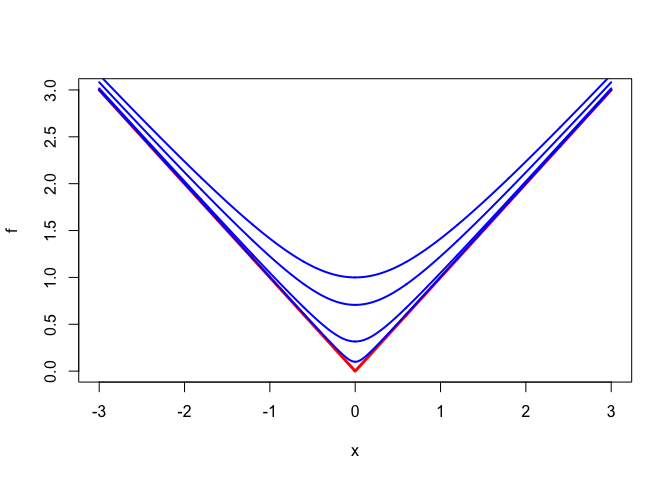Figure 1: Square root approximation: functipn

Clearly $$f_\epsilon(x)>|x|$$ for all $$x$$, and the maximum of $$f_\epsilon(x)-|x|$$ is $$\epsilon$$, attained at zero. Also $\lim_{x\rightarrow\infty} f_\epsilon(x)-|x|=\lim_{x\rightarrow-\infty} f_\epsilon(x) - |x| = 0.$

## 2.2 First Derivative

The first derivative is

$f'_\epsilon(x)=\frac{x}{\sqrt{x^2+\epsilon^2}}$ This is plotted, for the same values of $$\epsilon$$, in figure 2.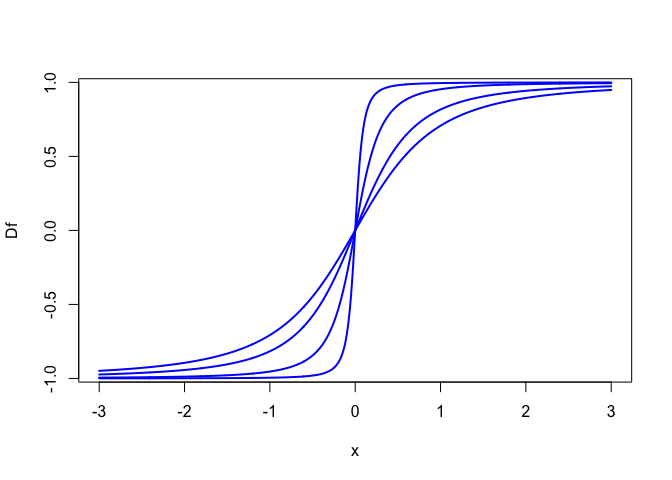Figure 2: Square root approximation: first derivative

We see that $$f'_\epsilon$$ is an increasing function of $$x$$, and thus $$f_\epsilon$$ is convex.

## 2.3 Second Derivative

The second derivative, plotted in figure 3, is

$f''_\epsilon(x)=\frac{1}{\sqrt{x^2+\epsilon^2}}\frac{\epsilon^2}{x^2+\epsilon^2}.$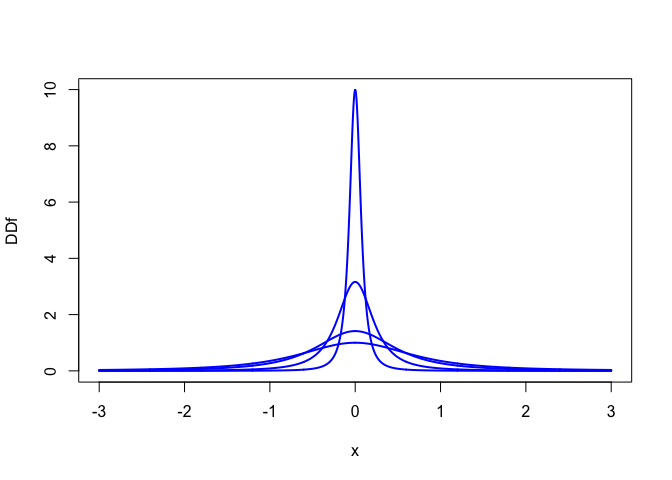Figure 3: Square root approximation: second derivative

The second derivative is non-negative, with the horizontal axis as its asymptote. It attains its maximum, equal to $$\epsilon^{-1}$$, at zero. This bound on the second derivative is the main reason for using $$\epsilon^2$$ in the definition of $$f_\epsilon$$.

# 3 Using convolution

## 3.1 Function

The following convolution approximation to $$|x|$$ was suggested by Voronin, Ozkaya, and Yoshida (2015). $\begin{equation}\label{E:g} g_\epsilon(x)\mathop{=}\limits^\Delta\frac{1}{\epsilon\sqrt{2\pi}}\int_{-\infty}^{+\infty}|x-y|\exp\{-\frac12\frac{y^2}{\epsilon^2}\}dy. \end{equation}$ Carrying out the integration gives $g_\epsilon(x)=x\left(2\Phi\left(\frac{x}{\epsilon}\right)-1\right)+2\epsilon\phi\left(\frac{x}{\epsilon}\right).$ Because $$g_\epsilon$$ is a weighted sum (integral) of a set of convex functions it is convex. We have $$g_\epsilon(x)>|x|$$, and the maximum of $$g_\epsilon(x)-|x|$$ is equal to $$\epsilon\sqrt{\frac{2}{\pi}}$$, attained at zero.

Voronin, Ozkaya, and Yoshida (2015) show that as $$\epsilon\rightarrow 0$$ we have both uniform and $$L_1$$ convergence of $$g_\epsilon$$ to $$|x|$$. Of course other convolution approximation with similar scale families (a.k.a. mollifiers), which have support converging to zero while maintaining mass equal to one, if scale goes to zero, can also be used.

Figure 4 show the approximation for $$\epsilon$$ equal to 1, .5, .1. and .01.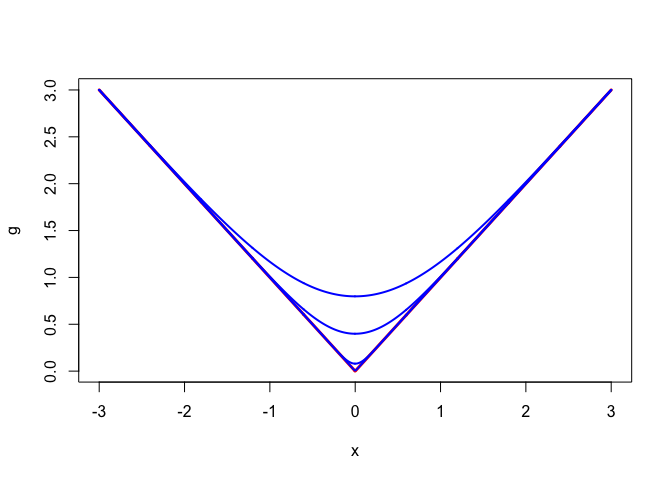Figure 4: Convolution approximation: function

## 3.2 First derivative

The first derivative, in figure 5 is

$g'_\epsilon(x)=2\Phi\left(\frac{x}{\epsilon}\right)-1.$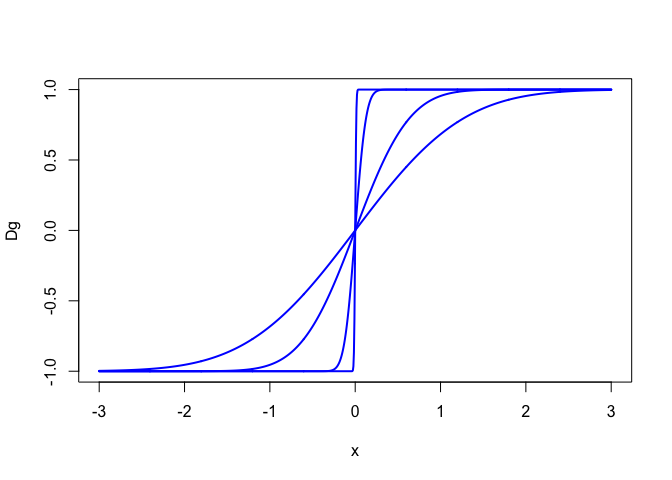Figure 5: Convolution approximation: first derivative

## 3.3 Second derivative

The second derivative, in figure 6, is $g''_\epsilon(x)=\frac{2}{\epsilon}\phi\left(\frac{x}{\epsilon}\right).$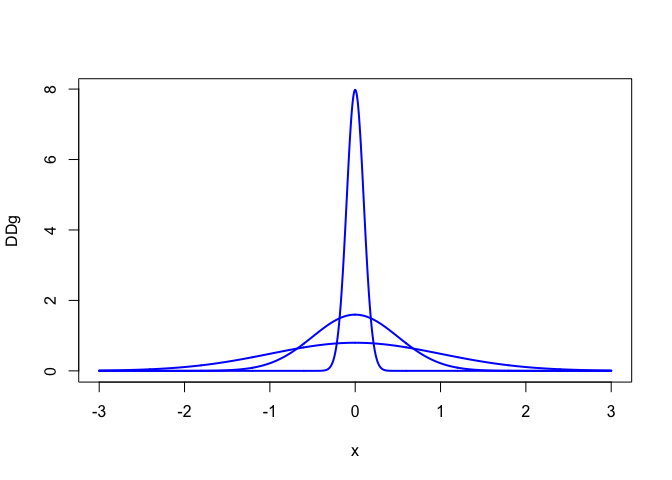Figure 6: Convolution approximation: second derivative

The second derivative is positive and unimodal, with a maximum equal to $$\frac{1}{\epsilon}\sqrt{\frac{2}{\pi}}$$ attained at zero.

# 4 Applications to $$\ell_1$$ Regression

Consider the problem of minimizing $\begin{equation}\label{E:l1loss} \sigma(\beta)=\sum_{i=1}^nw_i|y_i-x_i'\beta| \end{equation}$ This problem has been studied in statistics for many, many years. See, for example, Dielman (2005) or Farebrother (2013). Our contribution to the computational aspects of the problem is modest, because we are mainly interested in its connection to majorization theory.

## 4.1 AM/GM approach

Let’s start with the square root approximation $$f_\epsilon$$. The first majorization we construct is based on the AM/GM inequality. Because for each $$\beta,\tilde\beta$$ we have $\sqrt{\{(y_i-x_i'\beta)^2+\epsilon^2\}\{(y_i-x_i'\tilde\beta)^2+\epsilon^2\}} \leq\frac12\{(y_i-x_i'\beta)^2+\epsilon^2\}+\{(y_i-x_i'\tilde\beta)^2+\epsilon^2\},$ it follows that $\sum_{i=1}^nw_if_\epsilon(y_i-x_i'\beta)\leq\frac12\sum_{i=1}^nw_i\frac{1}{\sqrt{(y_i-x_i'\tilde\beta)^2+\epsilon^2}}\{(y_i-x_i'\beta)^2+(y_i-x_i'\tilde\beta)^2+2\epsilon^2\}.$ Thus if $$V(\beta)$$ is the diagonal matrix with diagonal elements $v_{ii}(\beta)=\frac{1}{\sqrt{(y_i-x_i'\beta)^2+\epsilon^2}}$ then majorization leads to an iteratively reweighted least squares algorithm. The update formula is $\beta^{(k+1)}=(X'WV(\beta^{(k)})X)^{-1}X'WV(\beta^{(k)})y.$

We apply this to the Boston housing data from the UCI repository (Newman et al. (1998)), loaded from the mlbench package (Leisch and Dimitriadou (2010)).

data("BostonHousing")
y <- BostonHousing$medv z <- BostonHousing[, 1:13] x <- cbind (1, apply(z, 2, as.numeric)) w <- rep (1, length(y)) The R function l1fn2() is used. h1 <- l1fn2 (x, y, aeps = 1e-2, itmax = 10000) We have convergence of function values, to a change of less than 10^{-10}, in 530 iterations. The output below gives both $$\sigma_\epsilon$$, the approximating loss function that we minimize, and $$\sigma$$ from $$\eqref{E:l1loss}$$, the actual $$\ell_1$$ loss function that we approximate. ## eps: 1.0e-03 itel: 530 sigma_e: 1559.812228 sigma: 1559.709732 ## beta: ## 14.633179 -0.144086 0.036871 0.019540 1.278130 -8.961015 5.324724 ## -0.030748 -1.035830 0.183490 -0.010219 -0.728994 0.011279 -0.300423 ## 4.2 Uniform quadratic majorization The second majorization uses an upper bound for the second derivative, as in Voss and Eckhardt (1980), Böhning and Lindsay (1988), Lange (2016), p. 100, or De Leeuw (2016), chapter 11. It can be applied both to our square root $$f_\epsilon$$ and convolution $$g_\epsilon$$ approximations. First the square root. Because $f_\epsilon(x)\leq f_\epsilon(y)+\frac{y}{\sqrt{y^2+\epsilon^2}}(x-y)+\frac{1}{2\epsilon}(x-y)^2$ we have $\sum_{i=1}^nw_if_\epsilon(y_i-x_i'\beta)\leq\sum_{i=1}^nw_if_\epsilon(y_i-x_i'\tilde\beta)-\sum_{i=1}^nw_i\left\{\frac{y_i-x_i'\tilde\beta}{\sqrt{(y_i-x_i'\tilde\beta)^2+\epsilon^2}}\right\}x_i'(\beta-\tilde\beta)+\frac{1}{2\epsilon}\sum_{i=1}^nw_i(x_i'(\beta-\tilde\beta))^2.$ which gives the majorization algorithm $\beta^{(k+1)}=(X'WX)^{-1}X'W(X\beta^{(k)}+\epsilon\ F_\epsilon'(\beta^{(k)})),$ where $$F_\epsilon'(\beta)$$ is a vector with elements $\mathcal{D}f_\epsilon(y_i-x_i'\beta)=\frac{y_i-x_i'\beta}{\sqrt{(y_i-x_i'\beta)^2+\epsilon^2}}.$ The corresponding expression for the convolution approximation $$g_\epsilon$$ is $\sum_{i=1}^nw_ig_\epsilon(y_i-x_i'\beta)\leq\sum_{i=1}^nw_ig_\epsilon(y_i-x_i'\tilde\beta)-\sum_{i=1}^nw_i\left\{2\Phi(\frac{y_i-x_i'\tilde\beta}{\epsilon})-1\right\}x_i'(\beta-\tilde\beta)+\frac12\frac{1}{\epsilon}\sqrt{\frac{2}{\pi}}\sum_{i=1}^nw_i(x_i'(\beta-\tilde\beta))^2.$ The majorization algorithm is $\beta^{(k+1)}=(X'WX)^{-1}X'W(X\beta^{(k)}+\epsilon\sqrt{\frac{\pi}{2}}G'_\epsilon(\beta^{(k)}))$ Given the shape of the second derivatives of the approximating functions, we expect this algorithm to converge slowly. The uniform upper bound is only good very close to the origin, and horribly bad everywhere else. The update function only makes a very small move in the descent direction. We illustrate the slow convergence with two analyses of the Boston housing data, one using the square root and one using the convolution approximation, using the R routines l1fu2() and l1gu2(). For the $$f_\epsilon$$ approximation we find h2 <- l1fu2 (x, y, aeps = 1e-2, itmax= 100000) ## eps: 1.0e-03 itel: 31800 sigma_e: 1559.812229 sigma: 1559.709719 ## beta: ## 14.636104 -0.144089 0.036873 0.019553 1.278381 -8.963905 5.324655 ## -0.030749 -1.035922 0.183485 -0.010218 -0.729064 0.011278 -0.300400 We see that convergence to our default precision requires 31800 iterations. The results for the convolution approximation are similar. h3 <- l1gu2 (x, y, aeps = 1e-2, itmax= 100000) ## eps: 1.0e-02 itel: 16848 sigma_e: 1559.744234 sigma: 1559.708984 ## beta: ## 14.780373 -0.144247 0.037000 0.020306 1.292046 -9.124745 5.323192 ## -0.030801 -1.041139 0.183103 -0.010149 -0.732826 0.011262 -0.299007 ## 4.3 Sharp quadratic majorization Uniform quadratic majorization fails rather miserably. But we have to realize that the AM/GM approach also leads to quadratic majorization, and in that case it works quite well. In order to improve the speed of convergence of the quadratic majorizations we turn to sharp non-uniform quadratic approximation, discussed in De Leeuw and Lange (2009). We use their theorem 4.5. Theorem 4.5. Suppose f(x) is an even, differentiable function on $$\mathbb{R}$$ such that the ratio $$f'(x)/x$$ is decreasing on $$(0,\infty)$$. Then the even quadratic $g(x)=\frac{f'(y)}{2y}(x^2-y^2)+f(y)$ is the best quadratic majorizer of $$f(x)$$ at the point $$y$$. Both approximations $$f_\epsilon$$ and $$g_\epsilon$$ satisfy the conditions of the theorem. For $$f_\epsilon$$ we find $\sqrt{x^2+\epsilon^2}\leq\frac12\frac{1}{\sqrt{y^2+\epsilon^2}}(x^2-y^2)+\sqrt{y^2+\epsilon^2}.$ Or $\sum_{i=1}^n w_if_\epsilon(y_i-x_i'\beta)\leq\sum_{i=1}^n w_if_\epsilon(y_i-x_i'\tilde\beta)+ \frac12\sum_{i=1}^n\frac{1}{\sqrt{(y_i-x_i'\tilde\beta)^2+\epsilon^2}}\{(y_i-x_i'\beta)^2-(y_i-x_i'\tilde\beta)^2\}.$ But this is exactly the majorization provided by the AM/GM approach. Consequently the AM/GM approach provides us with the sharpest quadratic majorization of $$f_\epsilon$$. We see that in the Boston housing example sharp quadratic majorization reduces the number of iterations from 31800 for uniform quadratic majorization to 530. The relation between AM/GM and sharp quadratic majorization is both new and interesting, but it does not give us an alternative algorithm. We can just use AM/GM and the R function l1f1(). For the convolution approximation $$g_\epsilon$$, however, the result in the theorem gives a new non-uniform quadratic majorization. From theorem 4.5 $g_\epsilon(x)\leq g_\epsilon(y)+\frac{2\Phi(\frac{y}{\epsilon})-1}{2y}(x^2-y^2),$ or $\sum_{i=1}^n w_ig_\epsilon(y_i-x_i'\beta)\leq\sum_{i=1}^n w_ig_\epsilon(y_i-x_i'\tilde\beta)+\frac12\sum_{i=1}^nw_i\frac{2\Phi(\frac{y_i-x_i'\tilde\beta}{\epsilon})-1}{y_i-x_i'\tilde\beta}\{(y_i-x_i'\beta)^2-(y_i-x_i'\tilde\beta)^2\}.$ We analyze the data with the R routine l1gn2(). h4 <- l1gn2 (x, y, aeps = 1e-2, itmax= 1000) ## eps: 1.0e-02 itel: 335 sigma_e: 1559.744234 sigma: 1559.708994 ## beta: ## 14.778534 -0.144244 0.036996 0.020294 1.291961 -9.123601 5.323291 ## -0.030799 -1.041089 0.183106 -0.010150 -0.732776 0.011262 -0.299028 For uniform quadratic majorization we needed 16848 iterations, for sharp majorization we only need 335. ## 4.4 Rate of Convergence Suppose $$\eta$$ is a majorization scheme for $$\tau$$, i.e. $$\eta:\mathbb{R}^n\otimes\mathbb{R}^n\rightarrow\mathbb{R}$$ and $$\tau:\mathbb{R}^n\rightarrow\mathbb{R}$$ such that $$\eta(x,y)\geq\tau(x)$$ for all $$x,y$$ and $$\eta(x,x)=\tau(x)$$ for all $$x$$. Also assume sufficient differentiability and uniqueness of the minima in majorization iterations. De Leeuw (1994) (also see De Leeuw (2016)) shows that the convergence rate of the majorization algorithm is the largest eigenvalue of the matrix $I-\{\mathcal{D}_{11}\eta(x,x)\}^{-1}\mathcal{D}^2\tau(x)=-\{\mathcal{D}_{11}\eta(x,x)\}^{-1}\mathcal{D}_{12}\eta(x,x).$ evaulated at the fixed point $$x$$. This is the Jacobian of the update function, that maps $$x^{(k)}$$ to its successor $$x^{(k+1)}$$. Note that in our majorization example $$\eta$$ is a quadratic in $$x$$. Thus the second derivatives $$\mathcal{D}_{11}$$ do not depend on $$x$$ and are simply the matrix of the quadratic form. If $$\sigma_\epsilon^f(\beta)\mathop{=}\limits^\Delta\sum_{i=1}^n w_if_\epsilon(y_i-x_i'\beta)$$ then $\mathcal{D}^2\sigma_\epsilon^f(\beta)=\epsilon^2\sum_{i=1}^n w_i\left\{(y_i-x_i'\beta)^2+\epsilon^2\right\}^{-\frac32}x_i^{\ }x_i'$ If $$\sigma_\epsilon^g(\beta)\mathop{=}\limits^\Delta\sum_{i=1}^n w_ig_\epsilon(y_i-x_i'\beta)$$ then $\mathcal{D}^2\sigma_\epsilon^g(\beta)=\frac{2}{\epsilon}\sum_{i=1}^n w_i\phi(\frac{y_i-x_i'\beta}{\epsilon})x_i^{\ }x_i'$ The function computeThatRate() gives the theoretical convergence rates at the $$\ell_1$$ solution for the Boston housing data for various values of $$\epsilon$$ and for all four quadratic majorizations (uniform-f, uniform-g, non-uniform-F, and non-uniform-g). epss <- c (5, 2, 1, .5, .1, .01, .005) beta <- h1$beta
computeThatRate (x, y, w, beta, epss)
##  5.0000000000 0.6576324826 0.5723976134 0.4279092359 0.3873481792
##  2.0000000000 0.8225953967 0.7835762713 0.5415263594 0.5286119029
##  1.0000000000 0.8963063025 0.8710636781 0.6125974817 0.5956478849
##  0.5000000000 0.9444461085 0.9288920175 0.7051830266 0.6940155591
##  0.1000000000 0.9889511967 0.9881274205 0.8800781159 0.9004846261
##  0.0100000000 0.9998468914 0.9999785172 0.9872466313 0.9985891937
##  0.0050000000 0.9999785958 0.9999999998 0.9964372125 0.9999999747

From the output we see that the convergence rate tends to one if $$\epsilon\downarrow 0$$. Thus asymptotically our quadratic majorization algorithms will have a sublinear convergence rate.

As we have already seen in our example, the non-uniform methods converge much faster than the uniform ones. Throughout this report we have chosen $$\epsilon$$ as some conventional numbers, without taking into account the scale of the data or the closeness of the fit. This is probably not a good idea, and especially in this large example our conventional $$\epsilon$$’s could very well be too small.

We check this more closely by using non-uniform majorization for seven different values of $$\epsilon$$. The results are below. Epsilon is in the first column, followed by the results for the number of iterations, the value of the approximation function at the minimum, and the value of the least abosolute deviation function itself.

##     5.000000   15.000000 3212.724906 1580.815597
##     2.000000   25.000000 2027.412038 1565.003116
##     1.000000   32.000000 1725.433167 1561.816194
##     0.500000   35.000000 1615.242147 1560.740384
##     0.100000   89.000000 1563.678895 1559.955323
##     0.050000  131.000000 1561.000966 1559.831086
##     0.010000  530.000000 1559.812228 1559.709732

For larger values of $$\epsilon$$ the values of $$\sigma(\beta)$$ and $$\sigma_\epsilon^f(\beta)$$ are very different. But the value of $$\sigma(\beta)$$ is already quite close to its minimum value, and that is of course what we are interested in. This is illustrated also in figure 7, where the 7 solutions for $$\beta$$ are plotted. Only the first one, for $$\epsilon$$ equal to 5, is any different, the rest are virtually indistinguishable. Thus if you want 10 decimals precision in your regression analysis then by all means choose $$\epsilon$$ small. But seeing a regression analysis with 10 decimals precision tell us more about the data analyst than it tells us about the data.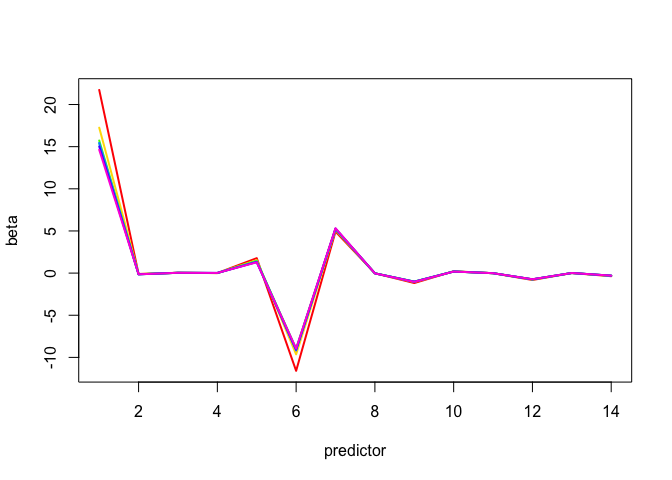Figure 7: Square root approximation: different epsilon

For completeness we repeat the analysis for the convolution approximation. The results are very much the same.

##     5.000000   13.000000 2660.097037 1589.022400
##     2.000000   22.000000 1815.279469 1565.920065
##     1.000000   27.000000 1634.626462 1561.803819
##     0.500000   32.000000 1580.827133 1560.899992
##     0.100000  110.000000 1560.955511 1560.006532
##     0.050000  123.000000 1560.122239 1559.837335
##     0.010000  335.000000 1559.744234 1559.708994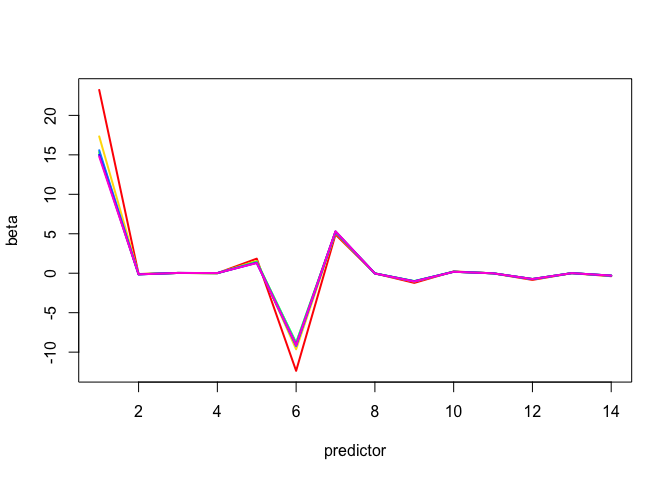Figure 8: Convolution approximation: different epsilon

# 5 Discussion

In future reports we may consider applications to nonlinear $$\ell_1$$, to the lasso, to support vector machines, and to unidimensional scaling.

# 6 Code

## 6.1 l1fu2.R

l1fu2 <-
function (x,
y,
w = rep(1, length (y)),
bold = NULL,
aeps = 1e-3,
eps = 1e-10,
itmax = 1000,
verbose = FALSE) {
ffunc <- function (x, y, b, aeps) {
z <- y - drop(x %*% b)
return (sqrt (z ^ 2 + aeps ^ 2))
}
fgrad <- function (x, y, b, aeps) {
z <- y - drop(x %*% b)
return (z / sqrt(z ^ 2 + aeps ^ 2))
}
if (is.null(bold)) {
bold <- lm.wfit (x, y, w)$coefficients } zold <- ffunc (x, y, bold, aeps) fold <- sum (w * zold) vold <- fgrad (x, y, bold, aeps) itel <- 1 repeat { bnew <- lm.wfit (x, drop(x %*% bold) + aeps * vold, w)$coefficients
znew <- ffunc (x, y, bnew, aeps)
fnew <- sum (w * znew)
vnew <- fgrad (x, y, bnew, aeps)
if (verbose)
cat(
"Iteration: ",
formatC(itel, width = 3, format = "d"),
"old: ",
formatC(
fold,
digits = 8,
width = 12,
format = "f"
),
"fnew: ",
formatC(
fnew,
digits = 8,
width = 12,
format = "f"
),
"\n"
)
if (((fold - fnew) < eps) || (itel == itmax))
break
vold <- vnew
fold <- fnew
bold <- bnew
itel <- itel + 1
}
ff <- sum (w * abs (y - drop (x %*% bnew)))
return(list(
itel = itel,
f = fnew,
ff = ff,
beta = bnew
))
}

## 6.2 l1fn2.R

l1fn2 <-
function (x,
y,
w = rep(1, length (y)),
bold = NULL,
aeps = 1e-3,
eps = 1e-10,
itmax = 1000,
verbose = FALSE) {
if (is.null(bold)) {
bold <- lm.wfit (x, y, w)$coefficients } zold <- sqrt ((y - drop (x %*% bold)) ^ 2 + aeps ^ 2) fold <- sum (w * zold) vold <- w / zold itel <- 1 repeat { bnew <- lm.wfit (x, y, vold)$coefficients
znew <- sqrt ((y - drop (x %*% bnew)) ^ 2 + aeps ^ 2)
fnew <- sum (w * znew)
vnew <- w / znew
if (verbose)
cat(
"Iteration: ",
formatC(itel, width = 3, format = "d"),
"old: ",
formatC(
fold,
digits = 8,
width = 12,
format = "f"
),
"fnew: ",
formatC(
fnew,
digits = 8,
width = 12,
format = "f"
),
"\n"
)
if (((fold - fnew) < eps) || (itel == itmax))
break
vold <- vnew
fold <- fnew
itel <- itel + 1
}
ff <- sum (w * abs (y - drop (x %*% bnew)))
return(list(
itel = itel,
f = fnew,
ff = ff,
beta = bnew
))
}

## 6.3 l1gu2.R

l1gu2 <-
function (x,
y,
w = rep(1, length (y)),
bold = NULL,
aeps = 1e-3,
eps = 1e-10,
itmax = 1000,
verbose = FALSE) {
cfunc <- function (x, y, b, aeps) {
z <- y - drop (x %*% b)
return (z * (2 * pnorm(z / aeps) - 1) + 2 * aeps * dnorm(z / aeps))
}
cgrad <- function (x, y, b, aeps) {
z <- y - drop(x %*% b)
return (2 * pnorm(z / aeps) - 1)
}
bnd <- aeps * sqrt (pi / 2)
if (is.null(bold)) {
bold <- lm.wfit (x, y, w)$coefficients } zold <- cfunc (x, y, bold, aeps) fold <- sum (w * zold) vold <- cgrad (x, y, bold, aeps) itel <- 1 repeat { bnew <- lm.wfit (x, drop(x %*% bold) + bnd * vold, w)$coefficients
znew <- cfunc (x, y, bnew, aeps)
fnew <- sum (w * znew)
vnew <- cgrad (x, y, bnew, aeps)
if (verbose)
cat(
"Iteration: ",
formatC(itel, width = 3, format = "d"),
"old: ",
formatC(
fold,
digits = 8,
width = 12,
format = "f"
),
"fnew: ",
formatC(
fnew,
digits = 8,
width = 12,
format = "f"
),
"\n"
)
if (((fold - fnew) < eps) || (itel == itmax))
break
vold <- vnew
fold <- fnew
bold <- bnew
itel <- itel + 1
}
ff <- sum (w * abs (y - drop (x %*% bnew)))
return(list(
itel = itel,
f = fnew,
ff = ff,
beta = bnew
))
}

## 6.4 l1gn2.R

l1gn2 <-
function (x,
y,
w = rep(1, length (y)),
bold = NULL,
aeps = 1e-3,
eps = 1e-10,
itmax = 1000,
verbose = FALSE) {
cfunc <- function (x, y, b, aeps) {
z <- y - drop (x %*% b)
return (z * (2 * pnorm(z / aeps) - 1) + 2 * aeps * dnorm(z / aeps))
}
cgrad <- function (x, y, b, aeps) {
z <- y - drop(x %*% b)
return (2 * pnorm(z / aeps) - 1)
}
if (is.null(bold)) {
bold <- lm.wfit (x, y, w)$coefficients } zold <- cfunc (x, y, bold, aeps) fold <- sum (w * zold) vold <- cgrad (x, y, bold, aeps) / (y - drop(x %*% bold)) itel <- 1 repeat { bnew <- lm.wfit (x, y, w * vold)$coefficients
znew <- cfunc (x, y, bnew, aeps)
fnew <- sum (w * znew)
vnew <- cgrad (x, y, bnew, aeps) / (y - drop(x %*% bnew))
if (verbose)
cat(
"Iteration: ",
formatC(itel, width = 3, format = "d"),
"old: ",
formatC(
fold,
digits = 8,
width = 12,
format = "f"
),
"fnew: ",
formatC(
fnew,
digits = 8,
width = 12,
format = "f"
),
"\n"
)
if (((fold - fnew) < eps) || (itel == itmax))
break
vold <- vnew
fold <- fnew
bold <- bnew
itel <- itel + 1
}
ff <- sum (w * abs (y - drop (x %*% bnew)))
return(list(
itel = itel,
f = fnew,
ff = ff,
beta = bnew
))
}

## 6.5 computeThatRate.R

computeThatRate <- function (x, y, w, beta, epss) {
for (eps in epss) {
r <- y - drop (x %*% beta)
v <- 1 / ((r ^ 2 + eps ^ 2) ^ (3 / 2))
d2_tau_f <- crossprod (x, v * w * x) * (eps ^ 2)
v <- dnorm (r / eps)
d2_tau_g <- (2 / eps) * crossprod (x, v * w * x)
d2_eta_f_uq <- crossprod (x, w * x) / eps
d2_eta_g_uq <- sqrt (2 / pi) * crossprod (x, w * x) / eps
v <- 1 / sqrt (r ^ 2 + eps ^ 2)
d2_eta_f_nq <- crossprod (x, w * v * x)
v <- (2 * pnorm (r / eps) - 1) / r
d2_eta_g_nq <- crossprod (x, w * v * x)
r_f_uq <-
1 - min (eigen (solve (d2_eta_f_uq, d2_tau_f))$values) r_g_uq <- 1 - min (eigen (solve (d2_eta_g_uq, d2_tau_g))$values)
r_f_nq <-
1 - min (eigen (solve (d2_eta_f_nq, d2_tau_f))$values) r_g_nq <- 1 - min (eigen (solve (d2_eta_g_nq, d2_tau_g))$values)
print (c(eps, r_f_uq, r_g_uq, r_f_nq, r_g_nq))
}
}